Want to share your content on R-bloggers? click here if you have a blog, or here if you don't.# What is a normal distribution?

The normal distribution is a function that defines how a set of measurements is distributed around the center of these measurements (i.e., the mean). Many natural phenomena in real life can be approximated by a bell-shaped frequency distribution known as the normal distribution or the Gaussian distribution.

The normal distribution is a mount-shaped, unimodal and symmetric distribution where most measurements gather around the mean. Moreover, the further a measure deviates from the mean, the lower the probability of occurring. In this sense, for a given variable, it is common to find values close to the mean, but less and less likely to find values as we move away from the mean. Last but not least, since the normal distribution is symmetric around its mean, extreme values in both tails of the distribution are equivalently unlikely. For instance, given that adult height follows a normal distribution, most adults are close to the average height and extremely short adults occur as infrequently as extremely tall adults.

In this article, the focus is on understanding the normal distribution, the associated empirical rule, its parameters and how to compute $$Z$$ scores to find probabilities under the curve (illustrated with examples). As it is a requirement in some statistical tests, we also show 4 complementary methods to test the normality assumption in R.

# Empirical rule

Data possessing an approximately normal distribution have a definite variation, as expressed by the following empirical rule:

• $$\mu \pm \sigma$$ includes approximately 68% of the observations
• $$\mu \pm 2 \cdot \sigma$$ includes approximately 95% of the observations
• $$\mu \pm 3 \cdot \sigma$$ includes almost all of the observations (99.7% to be more precise)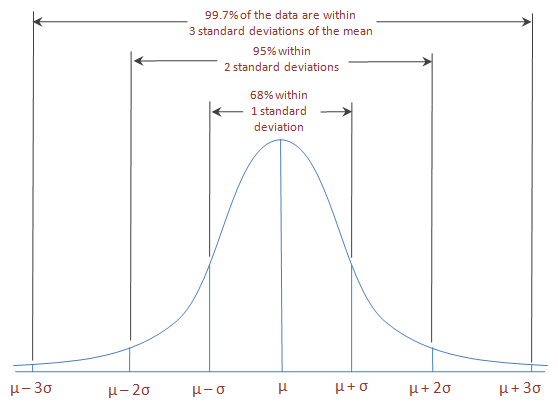Normal distribution & empirical rule (68-95-99.7% rule)

where $$\mu$$ and $$\sigma$$ correspond to the population mean and population standard deviation, respectively.

The empirical rule, also known as the 68-95-99.7% rule, is illustrated by the following 2 examples. Suppose that the scores of an exam in statistics given to all students in a Belgian university are known to have, approximately, a normal distribution with mean $$\mu = 67$$ and standard deviation $$\sigma = 9$$. It can then be deduced that approximately 68% of the scores are between 58 and 76, that approximately 95% of the scores are between 49 and 85, and that almost all of the scores (99.7%) are between 40 and 94. Thus, knowing the mean and the standard deviation gives us a fairly good picture of the distribution of scores. Now suppose that a single university student is randomly selected from those who took the exam. What is the probability that her score will be between 49 and 85? Based on the empirical rule, we find that 0.95 is a reasonable answer to this probability question.

The utility and value of the empirical rule are due to the common occurrence of approximately normal distributions of measurements in nature. For example, IQ, shoe size, height, birth weight, etc. are approximately normally-distributed. You will find that approximately 95% of these measurements will be within $$2\sigma$$ of their mean (Wackerly, Mendenhall, and Scheaffer 2014).

# Parameters

Like many probability distributions, the shape and probabilities of the normal distribution is defined entirely by some parameters. The normal distribution has two parameters: (i) the mean $$\mu$$ and (ii) the variance $$\sigma^2$$ (i.e., the square of the standard deviation $$\sigma$$). The mean $$\mu$$ locates the center of the distribution, that is, the central tendency of the observations, and the variance $$\sigma^2$$ defines the width of the distribution, that is, the spread of the observations.

The mean $$\mu$$ can take on any finite value (i.e., $$-\infty < \mu < \infty$$), whereas the variance $$\sigma^2$$ can assume any positive finite value (i.e., $$\sigma^2 > 0$$). The shape of the normal distribution changes based on these two parameters. Since there is an infinite number of combinations of the mean and variance, there is an infinite number of normal distributions, and thus an infinite number of forms.

For instance, see how the shapes of the normal distributions vary when the two parameters change: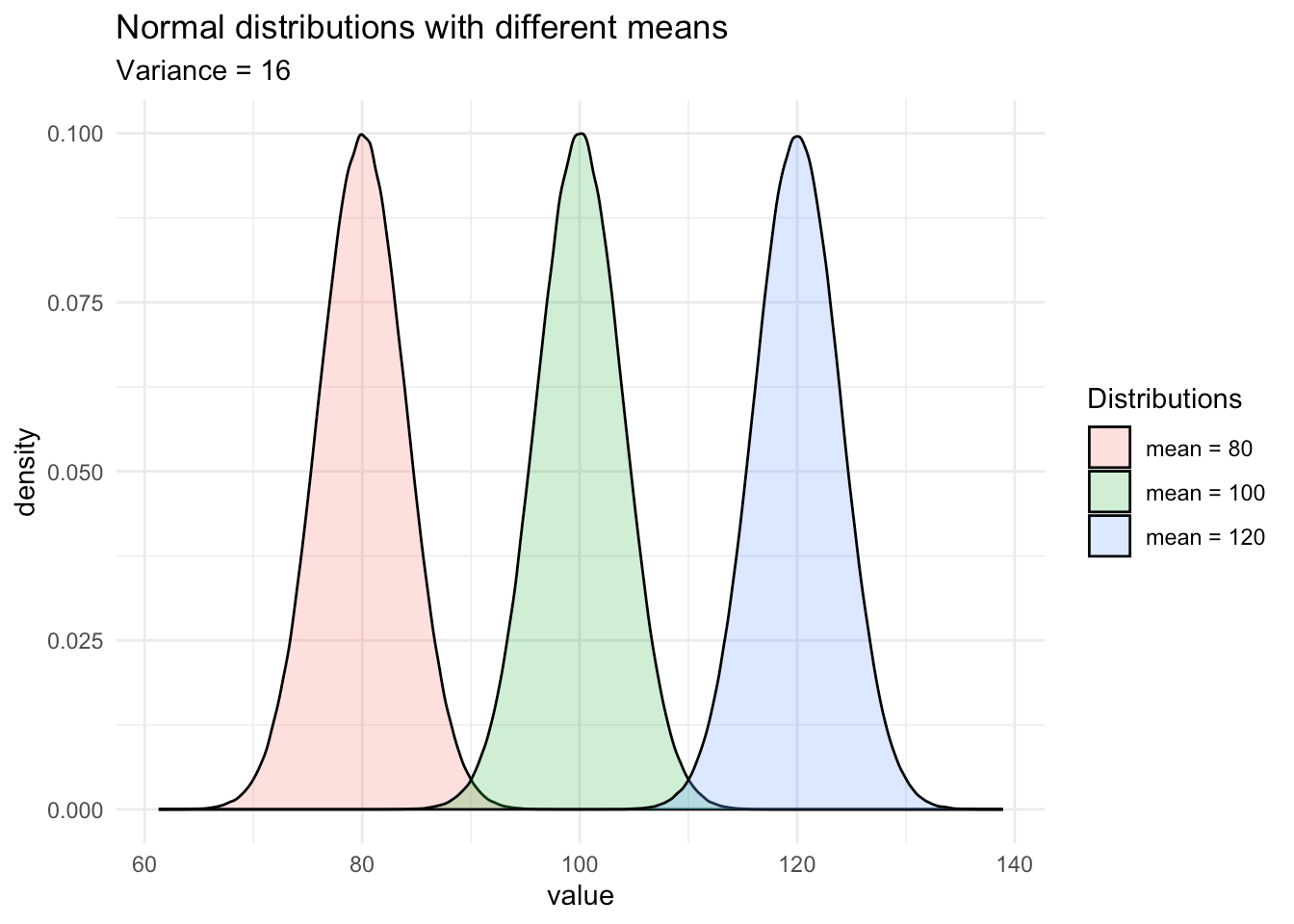As you can see on the second graph, when the variance (or the standard deviation) decreases, the observations are closer to the mean. On the contrary, when the variance (or standard deviation) increases, it is more likely that observations will be further away from the mean.

A random variable $$X$$ which follows a normal distribution with a mean of 430 and a variance of 17 is denoted $$X ~ \sim \mathcal{N}(\mu = 430, \sigma^2 = 17)$$.

We have seen that, although different normal distributions have different shapes, all normal distributions have common characteristics:

• They are symmetric, 50% of the population is above the mean and 50% of the population is below the mean
• The mean, median and mode are equal
• The empirical rule detailed earlier is applicable to all normal distributions

# Probabilities and standard normal distribution

Probabilities and quantiles for random variables with normal distributions are easily found using R via the functions pnorm() and qnorm(). Probabilities associated with a normal distribution can also be found using this Shiny app. However, before computing probabilities, we need to learn more about the standard normal distribution and the $$Z$$ score.

Although there are infinitely many normal distributions (since there is a normal distribution for every combination of mean and variance), we need only one table to find the probabilities under the normal curve: the standard normal distribution. The normal standard distribution is a special case of the normal distribution where the mean is equal to 0 and the variance is equal to 1. A normal random variable $$X$$ can always be transformed to a standard normal random variable $$Z$$, a process known as “scaling” or “standardization”, by subtracting the mean from the observation, and dividing the result by the standard deviation. Formally:

$Z = \frac{X – \mu}{\sigma}$

where $$X$$ is the observation, $$\mu$$ and $$\sigma$$ the mean and standard deviation of the population from which the observation was drawn. So the mean of the standard normal distribution is 0, and its variance is 1, denoted $$Z ~ \sim \mathcal{N}(\mu = 0, \sigma^2 = 1)$$.

From this formula, we see that $$Z$$, referred as standard score or $$Z$$ score, allows to see how far away one specific observation is from the mean of all observations, with the distance expressed in standard deviations. In other words, the $$Z$$ score corresponds to the number of standard deviations one observation is away from the mean. A positive $$Z$$ score means that the specific observation is above the mean, whereas a negative $$Z$$ score means that the specific observation is below the mean. $$Z$$ scores are often used to compare an individual to her peers, or more generally, a measurement compared to its distribution.

For instance, suppose a student scoring 60 at a statistics exam with the mean score of the class being 40, and scoring 65 at an economics exam with the mean score of the class being 80. Given the “raw” scores, one would say that the student performed better in economics than in statistics. However, taking into consideration her peers, it is clear that the student performed relatively better in statistics than in economics. Computing $$Z$$ scores allows to take into consideration all other students (i.e., the entire distribution) and gives a better measure of comparison. Let’s compute the $$Z$$ scores for the two exams, assuming that the score for both exams follow a normal distribution with the following parameters:

Statistics Economics
Mean 40 80
Standard deviation 8 12.5
Student’s score 60 65

$$Z$$ scores for:

• Statistics: $$Z_{stat} = \frac{60 – 40}{8} = 2.5$$
• Economics: $$Z_{econ} = \frac{65 – 80}{12.5} = -1.2$$

On the one hand, the $$Z$$ score for the exam in statistics is positive ($$Z_{stat} = 2.5$$) which means that she performed better than average. On the other hand, her score for the exam in economics is negative ($$Z_{econ} = -1.2$$) which means that she performed worse than average. Below an illustration of her grades in a standard normal distribution for better comparison: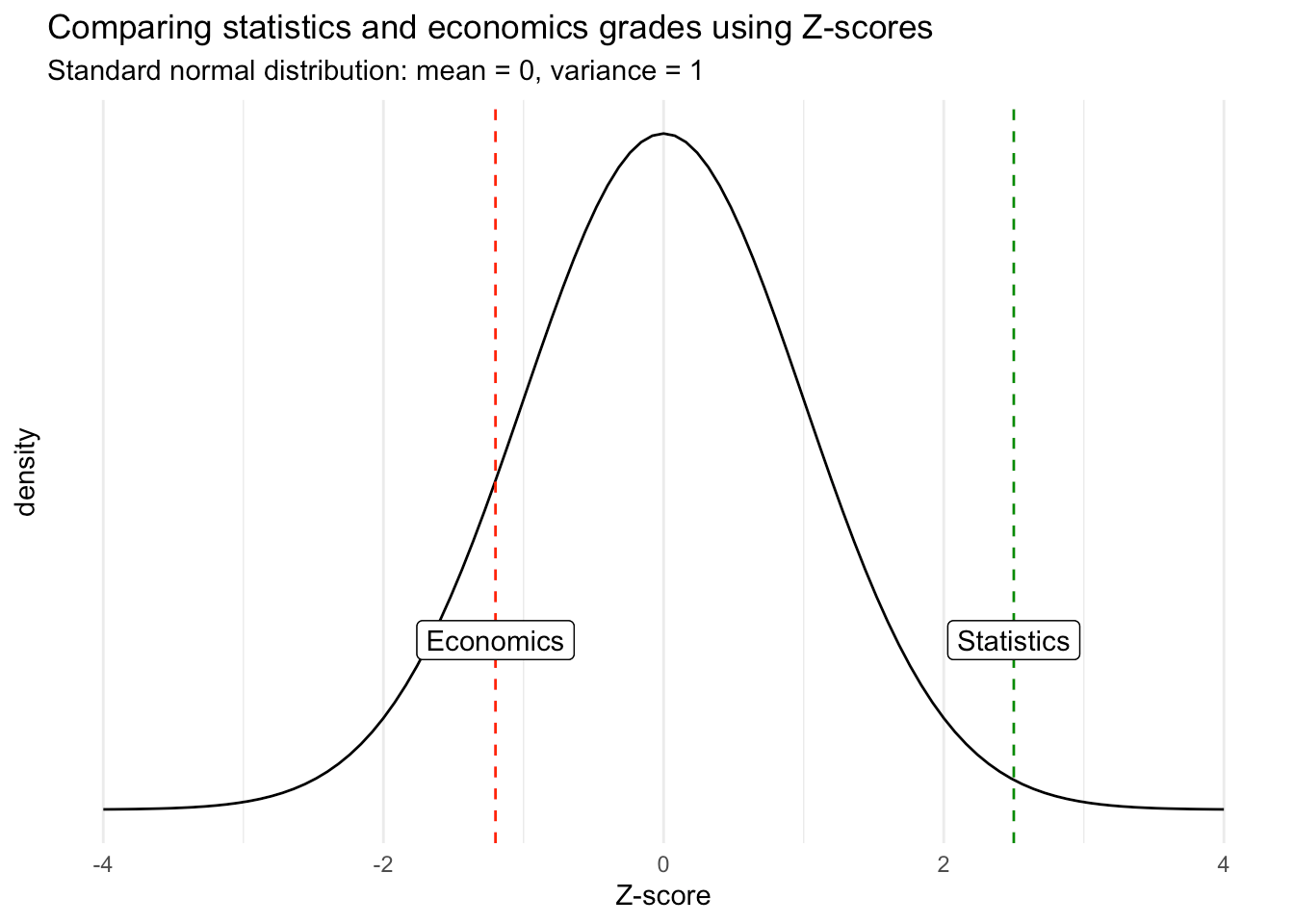Although the score in economics is better in absolute terms, the score in statistics is actually relatively better when comparing each score within its own distribution.

Furthermore, $$Z$$ score also enables to compare observations that would otherwise be impossible because they have different units for example. Suppose you want to compare a salary in € with a weight in kg. Without standardization, there is no way to conclude whether someone is more extreme in terms of her wage or in terms of her weight. Thanks to $$Z$$ scores, we can compare two values that were in the first place not comparable to each other.

Final remark regarding the interpretation of a $$Z$$ score: a rule of thumb is that an observation with a $$Z$$ score between -3 and -2 or between 2 and 3 is considered as a rare value. An observation with a $$Z$$ score smaller than -3 or larger than 3 is considered as an extremely rare value. A value with any other $$Z$$ score is considered as not rare nor extremely rare.

## Areas under the normal distribution in R and by hand

Now that we have covered the $$Z$$ score, we are going to use it to determine the area under the curve of a normal distribution.

Note that there are several ways to arrive at the solution in the following exercises. You may therefore use other steps than the ones presented to obtain the same result.

### Ex. 1

Let $$Z$$ denote a normal random variable with mean 0 and standard deviation 1, find $$P(Z > 1)$$.

We actually look for the shaded area in the following figure: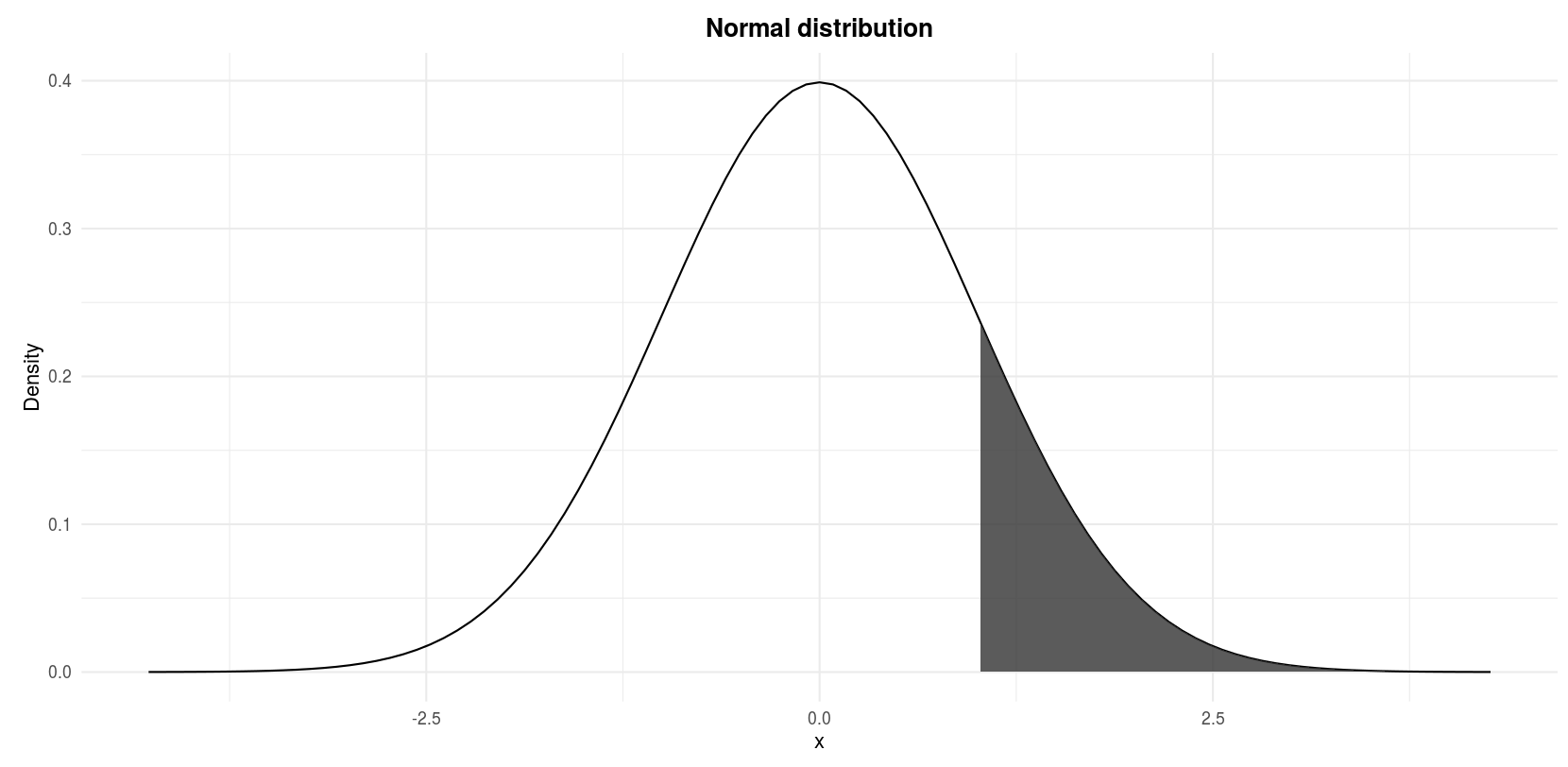Standard normal distribution: $$P(Z > 1)$$

#### In R

pnorm(1,
mean = 0,
sd = 1, # sd stands for standard deviation
lower.tail = FALSE
)
##  0.1586553

We look for the probability of $$Z$$ being larger than 1 so we set the argument lower.tail = FALSE. The default lower.tail = TRUE would give the result for $$P(Z < 1)$$. Note that $$P(Z = 1) = 0$$ so writing $$P(Z > 1)$$ or $$P(Z \ge 1)$$ is equivalent.

#### By hand

See that the random variable $$Z$$ has already a mean of 0 and a standard deviation of 1, so no transformation is required. To find the probabilities by hand, we need to refer to the standard normal distribution table shown below:Standard normal distribution table (Wackerly, Mendenhall, and Scheaffer 2014).

From the illustration at the top of the table, we see that the values inside the table correspond to the area under the normal curve above a certain $$z$$. Since we are looking precisely at the probability above $$z = 1$$ (since we look for $$P(Z > 1)$$), we can simply proceed down the first ($$z$$) column in the table until $$z = 1.0$$. The probability is 0.1587. Thus, $$P(Z > 1) = 0.1587$$. This is similar to what we found using R, except that values in the table are rounded to 4 digits.

### Ex. 2

Let $$Z$$ denote a normal random variable with mean 0 and standard deviation 1, find $$P(−1 \le Z \le 1)$$.

We are looking for the shaded area in the following figure: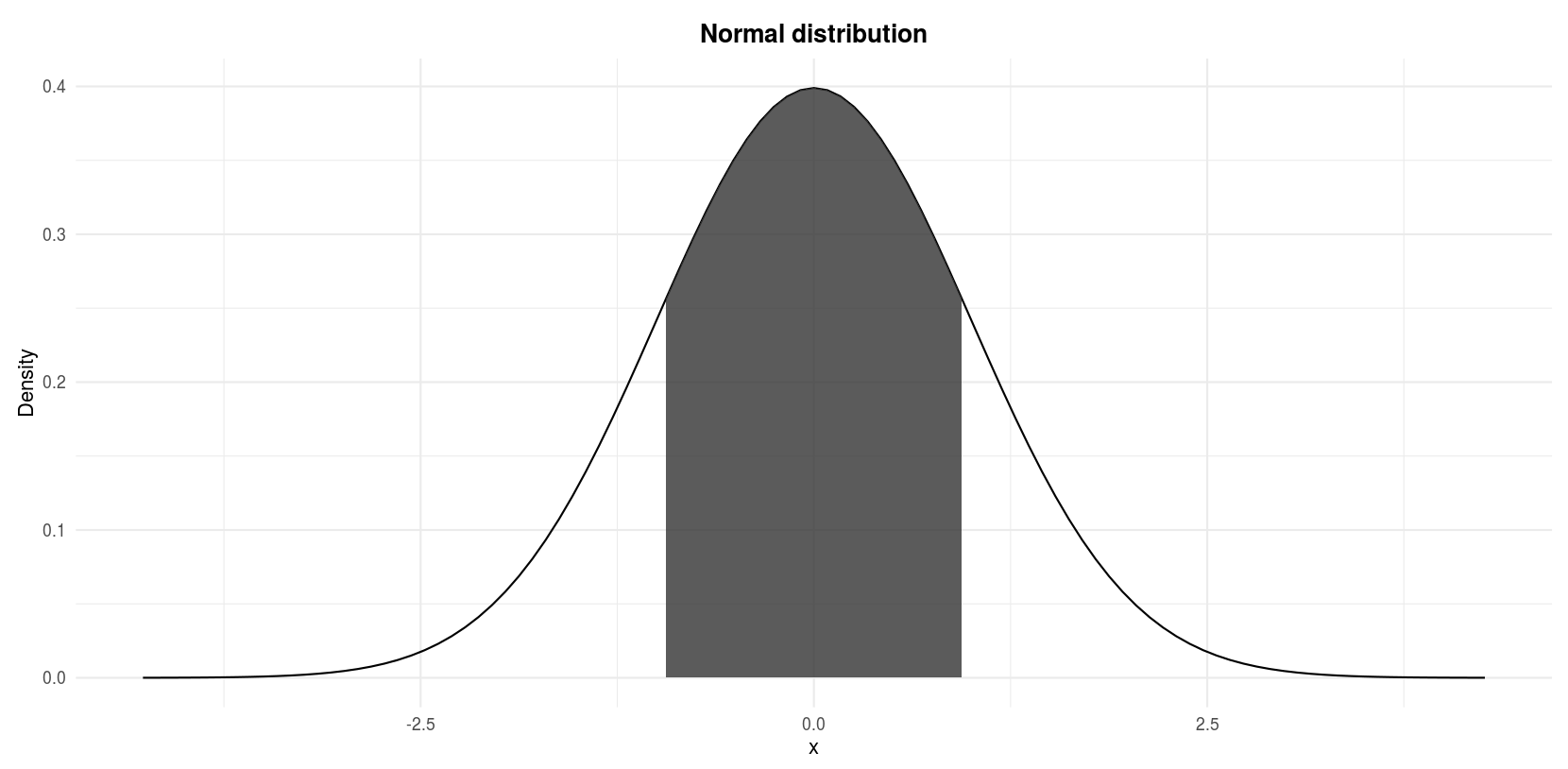Standard normal distribution: $$P(−1 \le Z \le 1)$$

#### In R

pnorm(1, lower.tail = TRUE) - pnorm(-1, lower.tail = TRUE)
##  0.6826895

Note that the arguments by default for the mean and the standard deviation are mean = 0 and sd = 1. Since this is what we need, we can omit them.1

#### By hand

For this exercise we proceed by steps:

1. The shaded area corresponds to the entire area under the normal curve minus the two white areas in both tails of the curve.
2. We know that the normal distribution is symmetric.
3. Therefore, the shaded area is the entire area under the curve minus two times the white area in the right tail of the curve, the white area in the right tail of the curve being $$P(Z > 1)$$.
4. We also know that the entire area under the normal curve is 1.
5. Thus, the shaded area is 1 minus 2 times $$P(Z > 1)$$:

$P(−1 \le Z \le 1) = 1 – 2 \cdot P(Z > 1)$ $= 1 – 2 \cdot 0.1587 = 0.6826$

where $$P(Z > 1) = 0.1587$$ has been found in the previous exercise.

### Ex. 3

Let $$Z$$ denote a normal random variable with mean 0 and standard deviation 1, find $$P(0 \le Z \le 1.37)$$.

We are looking for the shaded area in the following figure:Standard normal distribution: $$P(0 \le Z \le 1.37)$$

#### In R

pnorm(0, lower.tail = FALSE) - pnorm(1.37, lower.tail = FALSE)
##  0.4146565

#### By hand

Again we proceed by steps for this exercise:

1. We know that $$P(Z > 0) = 0.5$$ since the entire area under the curve is 1, half of it is 0.5.
2. The shaded area is half of the entire area under the curve minus the area from 1.37 to infinity.
3. The area under the curve from 1.37 to infinity corresponds to $$P(Z > 1.37)$$.
4. Therefore, the shaded area is $$0.5 – P(Z > 1.37)$$.
5. To find $$P(Z > 1.37)$$, proceed down the $$z$$ column in the table to the entry 1.3 and then across the top of the table to the column labeled .07 to read $$P(Z > 1.37) = .0853$$
6. Thus,

$P(0 \le Z \le 1.37) = P(Z > 0) – P(Z > 1.37)$ $= 0.5 – 0.0853 = 0.4147$

### Ex. 4

Recap the example presented in the empirical rule: Suppose that the scores of an exam in statistics given to all students in a Belgian university are known to have a normal distribution with mean $$\mu = 67$$ and standard deviation $$\sigma = 9$$. What fraction of the scores lies between 70 and 80?

We are looking for the shaded area in the following figure:$$P(70 \le X \le 80)$$ where $$X \sim \mathcal{N}(\mu = 67, \sigma^2 = 9^2)$$

#### In R

pnorm(70, mean = 67, sd = 9, lower.tail = FALSE) - pnorm(80, mean = 67, sd = 9, lower.tail = FALSE)
##  0.2951343

#### By hand

Remind that we are looking for $$P(70 \le X \le 80)$$ where $$X \sim \mathcal{N}(\mu = 67, \sigma^2 = 9^2)$$. The random variable $$X$$ is in its “raw” format, meaning that it has not been standardized yet since the mean is 67 and the variance is $$9^2$$. We thus need to first apply the transformation to standardize the endpoints 70 and 80 with the following formula:

$Z = \frac{X – \mu}{\sigma}$

After the standardization, $$x = 70$$ becomes (in terms of $$z$$, so in terms of deviation from the mean expressed in standard deviation):

$z = \frac{70 – 67}{9} = 0.3333$

and $$x = 80$$ becomes:

$z = \frac{80 – 67}{9} = 1.4444$

The figure above in terms of $$X$$ is now in terms of $$Z$$: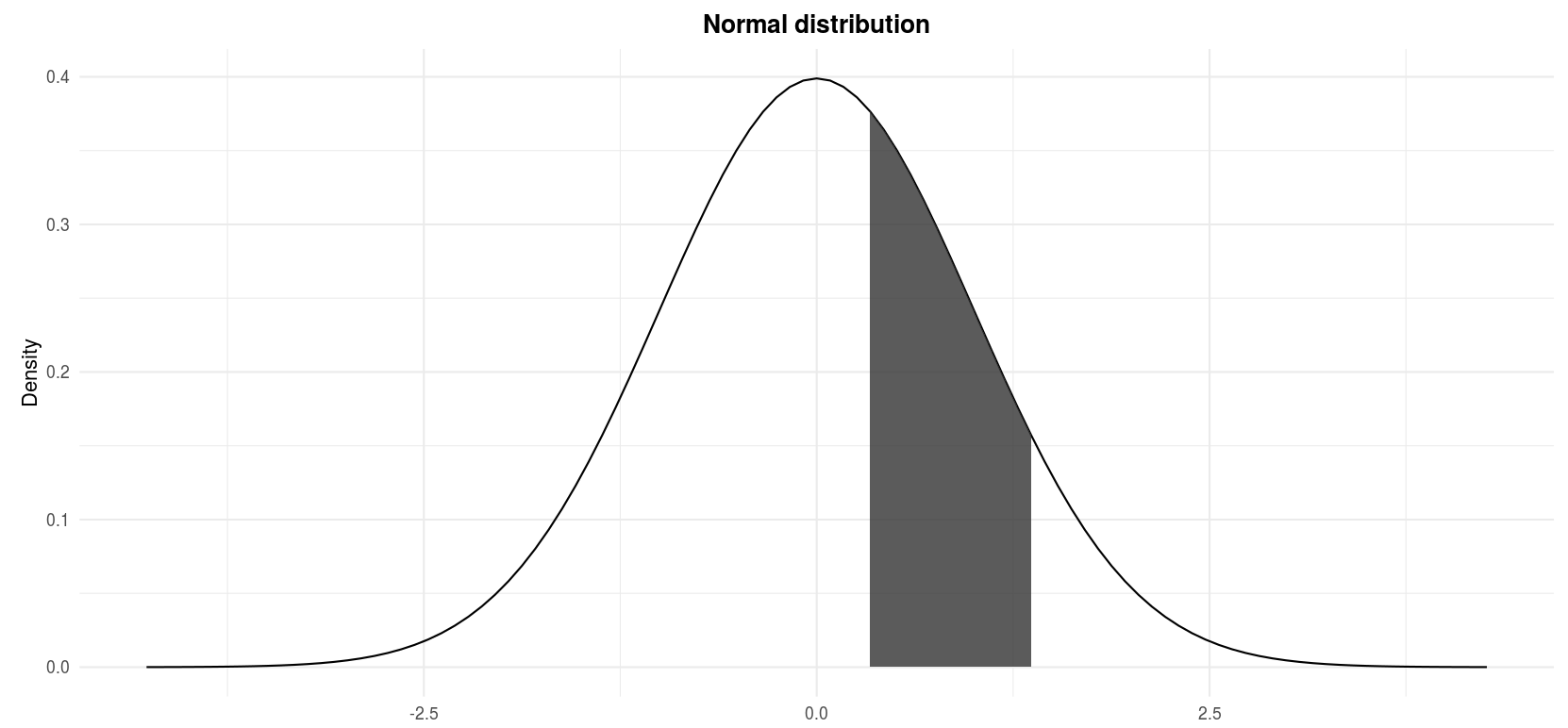$$P(0.3333 \le Z \le 1.4444)$$ where $$Z \sim \mathcal{N}(\mu = 0, \sigma^2 = 1)$$

Finding the probability $$P(0.3333 \le Z \le 1.4444)$$ is similar to exercises 1 to 3:

1. The shaded area corresponds to the area under the curve from $$z = 0.3333$$ to $$z = 1.4444$$.
2. In other words, the shaded area is the area under the curve from $$z = 0.3333$$ to infinity minus the area under the curve from $$z = 1.4444$$ to infinity.
3. From the table, $$P(Z > 0.3333) = 0.3707$$ and $$P(Z > 1.4444) = 0.0749$$
4. Thus:

$P(0.3333 \le Z \le 1.4444)$ $= P(Z > 0.3333) – P(Z > 1.4444)$ $= 0.3707 – 0.0749 = 0.2958$

The difference with the probability found using in R comes from the rounding.

To conclude this exercise, we can say that, given that the mean scores is 67 and the standard deviation is 9, 29.58% of the students scored between 70 and 80.

### Ex. 5

See another example in a context here.

# Why is the normal distribution so crucial in statistics?

The normal distribution is important for three main reasons:

• Some statistical hypothesis tests assume that the data follow a normal distribution
• The central limit theorem states that, for a large number of observations ($$n > 30$$), no matter what is the underlying distribution of the original variable, the distribution of the sample means ($$\overline{X}_n$$) and of the sum ($$S_n = \sum_{i = 1}^n X_i$$) may be approached by a normal distribution
• Linear and nonlinear regression assume that the residuals are normally-distributed

It is therefore useful to know how to test for normality in R, which is the topic of next sections.

# How to test the normality assumption

As mentioned above, some statistical tests require that the data follow a normal distribution, or the result of the test may be flawed.

In this section, we show 4 complementary methods to determine whether your data follow a normal distribution in R.

## Histogram

A histogram displays the spread and shape of a distribution, so it is a good starting point to evaluate normality. Let’s have a look at the histogram of a distribution that we would expect to follow a normal distribution, the height of 1,000 adults in cm:The normal curve with the corresponding mean and variance has been added to the histogram. The histogram follows the normal curve so the data seems to follow a normal distribution.

Below the minimal code for a histogram in R with the dataset iris:

data(iris)
hist(iris$Sepal.Length)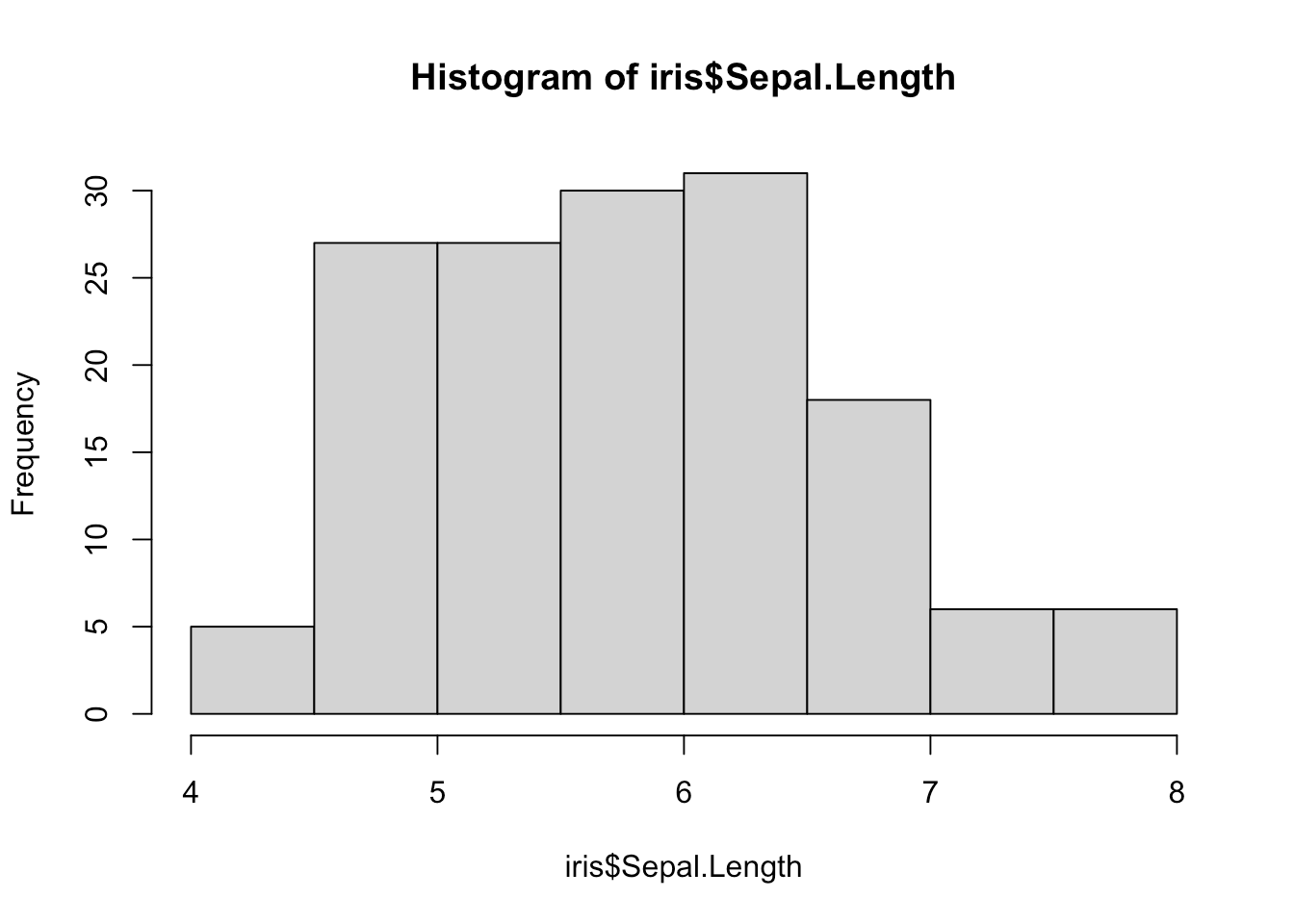ggplot(iris) + aes(x = Sepal.Length) + geom_histogram()Histograms are however not sufficient, particularly in the case of small samples because the number of bins greatly change its appearance. Histograms are not recommended when the number of observations is less than 20 because it does not always correctly illustrate the distribution. See two examples below with dataset of 10 and 12 observations: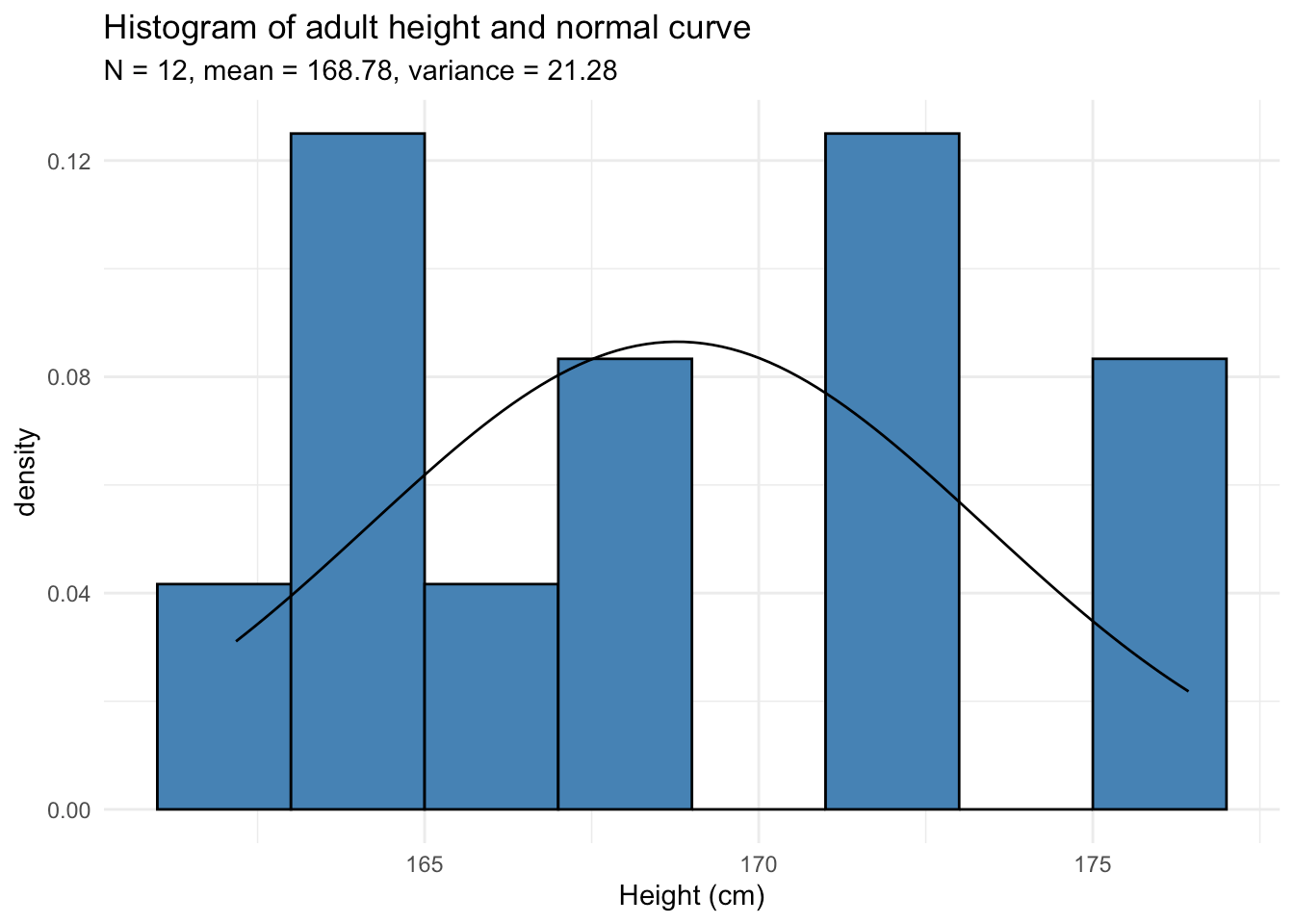Can you tell whether these datasets follow a normal distribution? Surprisingly, both follow a normal distribution! In the remaining of the article, we will use the dataset of the 12 adults. If you would like to follow my code in your own script, here is how I generated the data: set.seed(42) dat_hist <- data.frame( value = rnorm(12, mean = 165, sd = 5) ) The rnorm() function generates random numbers from a normal distribution (12 random numbers with a mean of 165 and standard deviation of 5 in this case). These 12 observations are then saved in the dataset called dat_hist under the variable value. Note that set.seed(42) is important to obtain the exact same data as me.2 ## Density plot Density plots also provide a visual judgment about whether the data follow a normal distribution. They are similar to histograms as they also allow to analyze the spread and the shape of the distribution. However, they are a smoothed version of the histogram. Here is the density plot drawn from the dataset on the height of the 12 adults discussed above: plot(density(dat_hist$value))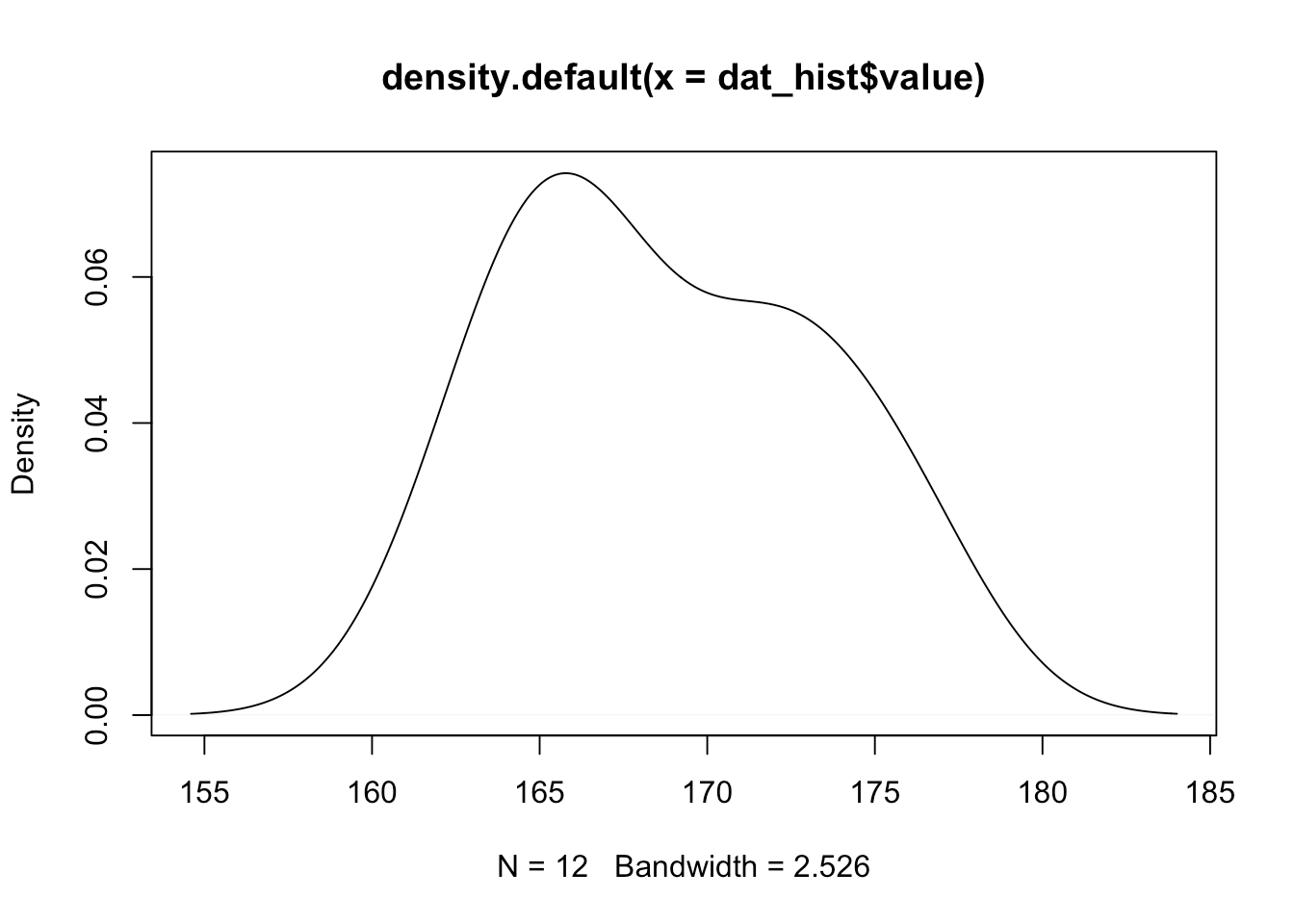In {ggpubr}:

library("ggpubr") # package must be installed first
ggdensity(dat_hist$value, main = "Density plot of adult height", xlab = "Height (cm)" )Since it is hard to test for normality from histograms and density plots only, it is recommended to corroborate these graphs with a QQ-plot. QQ-plot, also known as normality plot, is the third method presented to evaluate normality. ## QQ-plot Like histograms and density plots, QQ-plots allow to visually evaluate the normality assumption. Here is the QQ-plot drawn from the dataset on the height of the 12 adults discussed above: library(car) qqPlot(dat_hist$value)##  12  2

In {ggpubr}:

library(ggpubr)
ggqqplot(dat_hist$value)Instead of looking at the spread of the data (as it is the case with histograms and density plots), with QQ-plots we only need to ascertain whether the data points follow the line (sometimes referred as Henry’s line). If points are close to the reference line and within the confidence bands, the normality assumption can be considered as met. The bigger the deviation between the points and the reference line and the more they lie outside the confidence bands, the less likely that the normality condition is met. The height of these 12 adults seem to follow a normal distribution because all points lie within the confidence bands. When facing a non-normal distribution as shown by the QQ-plot below (systematic departure from the reference line), the first step is usually to apply the logarithm transformation on the data and recheck to see whether the log-transformed data are normally distributed. Applying the logarithm transformation can be done with the log() function.Note that QQ-plots are also a convenient way to assess whether residuals from regression analysis follow a normal distribution. ## Normality test The 3 tools presented above were a visual inspection of the normality. Nonetheless, visual inspection may sometimes be unreliable so it is also possible to formally test whether the data follow a normal distribution with statistical tests. These normality tests compare the distribution of the data to a normal distribution in order to assess whether observations show an important deviation from normality. The two most common normality tests are Shapiro-Wilk’s test and Kolmogorov-Smirnov test. Both tests have the same hypotheses, that is: • $$H_0$$: the data follow a normal distribution • $$H_1$$: the data do not follow a normal distribution Shapiro-Wilk test is recommended for normality test as it provides better power than Kolmogorov-Smirnov test.3 In R, the Shapiro-Wilk test of normality can be done with the function shapiro.test():4 shapiro.test(dat_hist$value)
##
##  Shapiro-Wilk normality test
##
## data:  dat_hist\$value
## W = 0.93968, p-value = 0.4939

From the output, we see that the $$p$$-value $$> 0.05$$ implying that we do not reject the null hypothesis that the data follow a normal distribution. This test goes in the same direction than the QQ-plot, which showed no significant deviation from the normality (as all points lied within the confidence bands).

It is important to note that, in practice, normality tests are often considered as too conservative in the sense that for large sample size ($$n > 50$$), a small deviation from the normality may cause the normality condition to be violated. A normality test is a hypothesis test, so as the sample size increases, their capacity of detecting smaller differences increases. So as the number of observations increases, the Shapiro-Wilk test becomes very sensitive even to a small deviation from normality. As a consequence, it happens that according to the normality test the data do not follow a normal distribution although the departures from the normal distribution are negligible and the data in fact follow a normal distribution. For this reason, it is often the case that the normality condition is verified based on a combination of all methods presented in this article, that is, visual inspections (with histograms and QQ-plots) and a formal inspection (with the Shapiro-Wilk test for instance).

I personally tend to prefer QQ-plots over histograms and normality tests so I do not have to bother about the sample size. This article showed the different methods that are available, your choice will of course depends on the type of your data and the context of your analyses.

Thanks for reading. I hope the article helped you to learn more about the normal distribution and how to test for normality in R.

As always, if you have a question or a suggestion related to the topic covered in this article, please add it as a comment so other readers can benefit from the discussion.

1. The argument lower.tail = TRUE is also the default so we could omit it as well. However, for clarity and to make sure I compute the propabilities in the correct side of the curve, I used to keep this argument explicit by writing it.↩︎

2. The set.seed() function accepts any numeric as argument. Generating random numbers (via rnorm() for instance) implies that R will generates different random numbers every time you generate these random numbers (so every time you run the function rnorm()). To make sure R generates the exact same numbers every time you run the function, a seed can be set with the function set.seed(). Setting a seed implies that R will generate random numbers, but these numbers will always be the same as long as the seed is the same. This allows to replicate results that are based on a random generation. Change the seed if you want to generate other random values.↩︎

3. The Shapiro-Wilk test is based on the correlation between the sample and the corresponding normal scores.↩︎

4. In R, the Kolmogorov-Smirnov test is performed with the function ks.test().↩︎